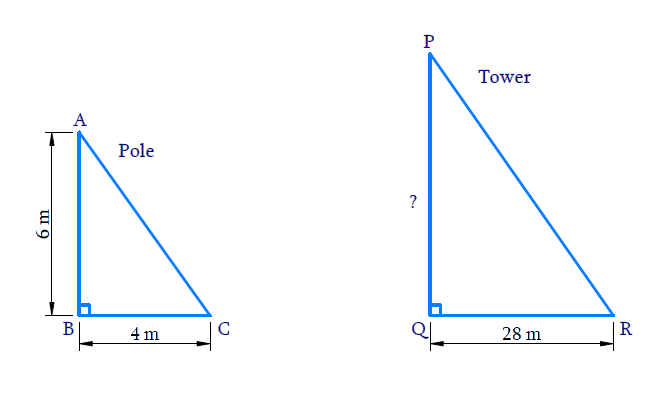# Ex.6.3 Q15 Triangles Solution - NCERT Maths Class 10

Go back to  'Ex.6.3'

## Question

A vertical pole of length $$6$$ $$\rm m$$ casts a shadow $$4$$ $$\rm m$$ long on the ground and at the same time a tower casts a shadow $$28$$ $$\rm m$$ long. Find the height of the tower.

DiagramVideo Solution
Triangles
Ex 6.3 | Question 15

## Text Solution

Reasoning:

The ratio of any two corresponding sides in two equiangular triangles is always the same.

Steps:

$$AB$$ is the pole $$=$$ $$6$$ $$\rm m$$

$$BC$$ is the shadow of pole $$=$$ $$4$$ $$\rm m$$

$$PQ$$ is the tower $$=?$$

$$QR$$ is the shadow of the tower $$=$$ $$28$$ $$\rm m$$

In $$\Delta ABD$$ and $$\Delta PQR$$

\begin{align}&\angle ABC=\angle PQR= 90^{\circ} \\ &\begin{bmatrix}\text{The objects and shadow are } \\ \text{perpendicular to each other}\end{bmatrix}\end{align}

\begin{align}&\angle BAC=\angle QPR \\ &\begin{bmatrix} \text{Sunrays fall on the }\\\text{pole and tower at the }\\ \text{same angle, at the same time} \end{bmatrix} \end{align}

\begin{align}\Rightarrow \quad \Delta ABC \sim \Delta PQR \\ \text{(AA Criterion)} \end{align}

The ratio of any two corresponding sides in two equiangular triangles is always the same.

\begin{align} \Rightarrow\qquad \frac{A B}{B C}&=\frac{P Q}{Q R} \\ \frac{6 \rm {}m}{6 \rm{}m}&=\frac{P Q}{28\rm{} m} \\ \Rightarrow\quad P Q&=\frac{6 \times 28}{4}{\rm {m}} \\ P Q &=42\rm{} m \end{align}

Hence, the height of the tower is $$42 \rm{}\,m.$$

Learn from the best math teachers and top your exams

• Live one on one classroom and doubt clearing
• Practice worksheets in and after class for conceptual clarity
• Personalized curriculum to keep up with school